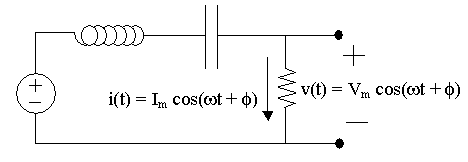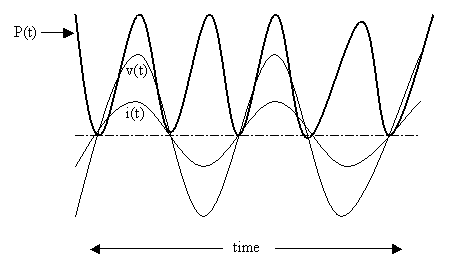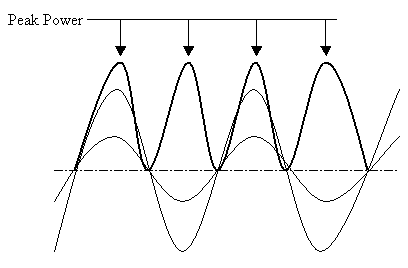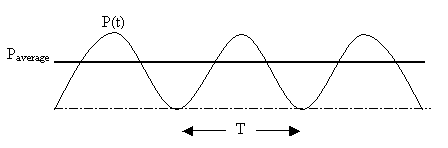AC Power
Calculating Power for AC circuits and the different forms of Power

 Outline: Instantaneous Power Peak Power Average Power Calculating Average Power Root Mean Square (RMS) values

Instantaneous Power   -  P(t)

Instantaneous Power is the rate at which energy is absorbed/delievered
for an element at a specific time.

Instantaneous power varies with time.

P(t) = v(t)*i(t)

P(t) = Vmcos(ωt + φ)*Imcos(ωt + θ)

 To find the power absorbed in the resistor we would have to multiply voltage times current. Since both voltage and current are sinusoidal, instantaneous power would also be sinusoidal. To find instantaneous power at a specific time requires plugging in a specific 't'.Here are some hypothetical waveforms: P(t) varies with timeSummary

Instantaneous Power is a function of time. It is not a constant.

It is not an average, therefore it is not used for billing utility customers.

Top of Page

Peak Power

Peak Power is the maximum instantaneous power. Peak Power
is an important specification used to characterize a device/element.
If the power absorbed by an element should exceed the Peak Power,
then the device may be permanently damaged.

Thus Peak Power is a spec used during the design phase by engineers.

 Here Peak Power is indicated on the waveform:Summary

Peak Power is used to characterize a device.
This information is usually found in a Parts Data Book published
by a device manufacturer such as Intel or AMD.

Top of Page

Average Power

Average Power is the average rate at which energy
is absorbed. Average Power is independent of time.
It is used by utility companies for billing purposes.

Recall Instantaneous Power:    P(t) = v(t)*i(t)

Paverage = (1/T) P(t) dt

where T is the period and P(t) is integrated over a period.

 Average Power is not a function of time:Let v(t) = Vmcos(ωt + φ)
and Let i(t) = Imcos(ωt + φ - θ)

Paverage = (ω/2π) ∫ [Vmcos(ωt + φ) * Imcos(ωt + φ - θ)]

(Note that T = 2π/ω)

If the above equation is simplifed, one gets the following result:

Paverage = ½(VmIm)cos(θ)

Note that θ = φ - (φ - θ)     OR     θ = Phase of v(t) - Phase of i(t)

Summary

Average Power is calcuated by multiplying the magnitudes Vm and Im and then dividing by 2.
This product is multiplied by the cosine of the the angle at which voltage leads current.

What is the average power for a resistor?

Since voltage and current are in phase across a resistor, then:
Paverage = ½(VmIm)
because cos(0) = 1

What is the average power for a capacitor?

Since voltage lags current by 90o across a capacitor, then:
Paverage = ½(VmIm) cos(-90o) = 0

What is the average power for a inductor?

Since voltage leads current by 90o across an inductor, then:
Paverage = ½(VmIm) cos(90o) = 0

This should make sense since capacitors and inductors are storage elements.
They store and release energy. They do not absorb energy.

Top of Page

Calculating Average Power

Alternate forms to Paverage:

Recall that Paverage = ½(VmIm)cos(θ)

Note that Z = V/I   where Z is impedance.

Putting this equation in phasor form:
Z = (Vm  :  Phase of v(t)) / (Im  :  Phase of i(t))

|Z| = Vm/Im     and     Angle of Z = Phase of v(t) - Phase of i(t)

We must say angle of Z not phase of Z because Z (unlike voltage and current) is NOT a phasor/sinusoidal!

VmIm = |Z| Im²
θ = Phase of v(t) - Phase of i(t)

Therefore an alternate to Paverage is:

Paverage = ½ |Z| Im² cos (Angle of Z)cos (Angle of Z) = Real(Z) / |Z|

Paverage = ½ |Z| Im² Real(Z) / |Z|

Paverage = ½ Im² Real(Z)

The real part of Z is the resistive portion of the impedance. Note that
reactive components such as capacitors and inductors do not contribute to
the average power absorbed.

## Study Problems

After clicking on the following link enter 11-1 for the problem and 1 for the step:
Study Problem 11-1
After clicking on the following link enter 11-2 for the problem and 1 for the step:
Study Problem 11-2
After clicking on the following link enter 11-3 for the problem and 1 for the step:
Study Problem 11-3

Top of Page

Root Mean Square (RMS) values

An RMS value is the DC equivalent current or voltage that would deliver
the same average power as the AC current or voltage.

Recall that power in a DC circuit is:
P = VI = I² R

To find IRMS:

IRMS ² R = 1/T ∫ R i(t)² dt = Paverage

Canceling out the R we get:

IRMS ² = 1/T ∫ i(t)² dt

If this equation is simplified (left to the more advanced student) we get:

IRMS = Im/√2

Similarly we get:

VRMS = Vm/√2

We now have other equations for calculating average power:

Paverage = VRMS IRMS cos (θ)

Paverage = IRMS² Real(Z)

When we see our household voltage source expressed as:
120 Volts - 60 Hz
this is really an RMS voltage value.

To find the peak voltage Vm we must multiply by √2:
Vpeak = 120V * √2 = 170 Volts

## Study Problems

After clicking on the following link enter 11-4 for the problem and 1 for the step:
Study Problem 11-4

Top of Page

Back To Index